# NLP教程(3) | 神经网络与反向传播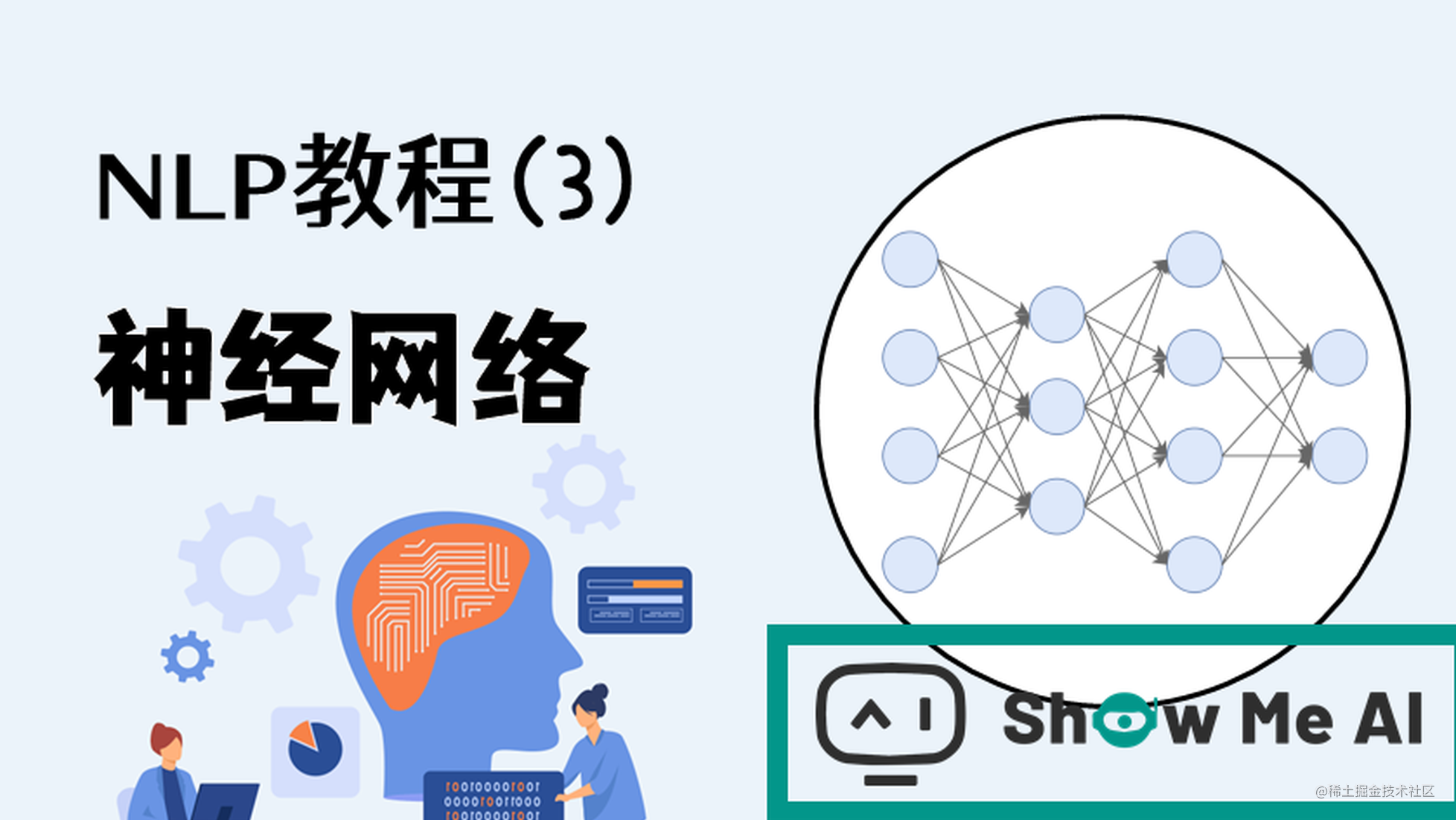本系列为斯坦福CS224n《自然语言处理与深度学习(Natural Language Processing with Deep Learning)》的全套学习笔记，对应的课程视频可以在 这里 查看。ShowMeAI为CS224n课程的全部课件，做了中文翻译和注释，并制作成了 GIF动图！点击 第3讲-词向量进阶第4讲-神经网络反向传播与计算图 查看的课件注释与带学解读。更多资料获取方式见文末。

# 引言

CS224n是顶级院校斯坦福出品的深度学习与自然语言处理方向专业课程，核心内容覆盖RNN、LSTM、CNN、transformer、bert、问答、摘要、文本生成、语言模型、阅读理解等前沿内容。

• 神经网络
• 反向传播
• 梯度计算
• 神经元
• 合页损失
• 梯度检查
• Xavier参数初始化
• 学习率

# 1.神经网络基础

（本部分内容也可以参考ShowMeAI的对吴恩达老师课程的总结文章深度学习教程 | 神经网络基础深度学习教程 | 浅层神经网络深度学习教程 | 深层神经网络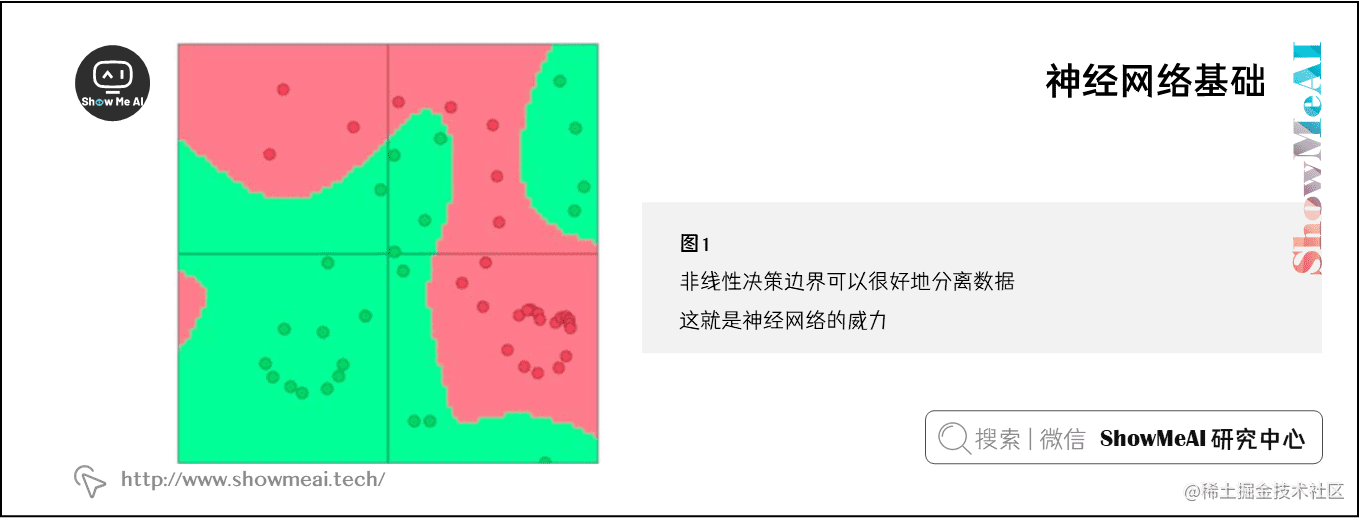## 1.1 单个神经元

$a=\frac{1}{1+exp(-(w^{T}x+b))}$

$a=\frac{1}{1+exp(-[w^{T}\;\;x]\cdot [x\;\;1])}$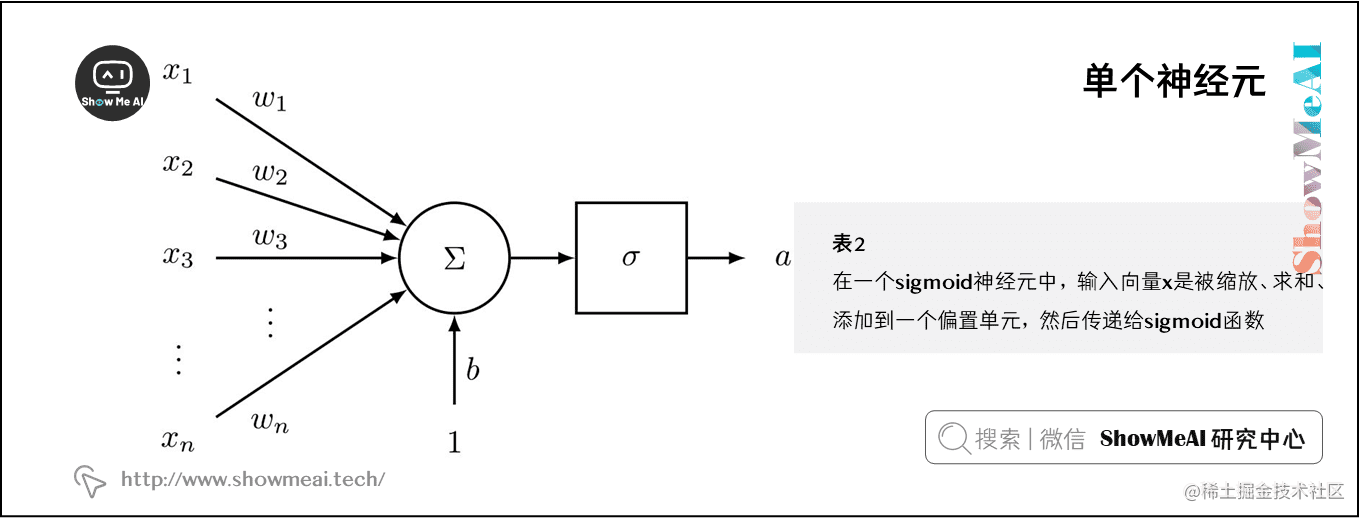❐ 神经元是神经网络的基本组成部分。我们将看到神经元可以是许多允许非线性在网络中积累的函数之一。

## 1.2 单层神经网络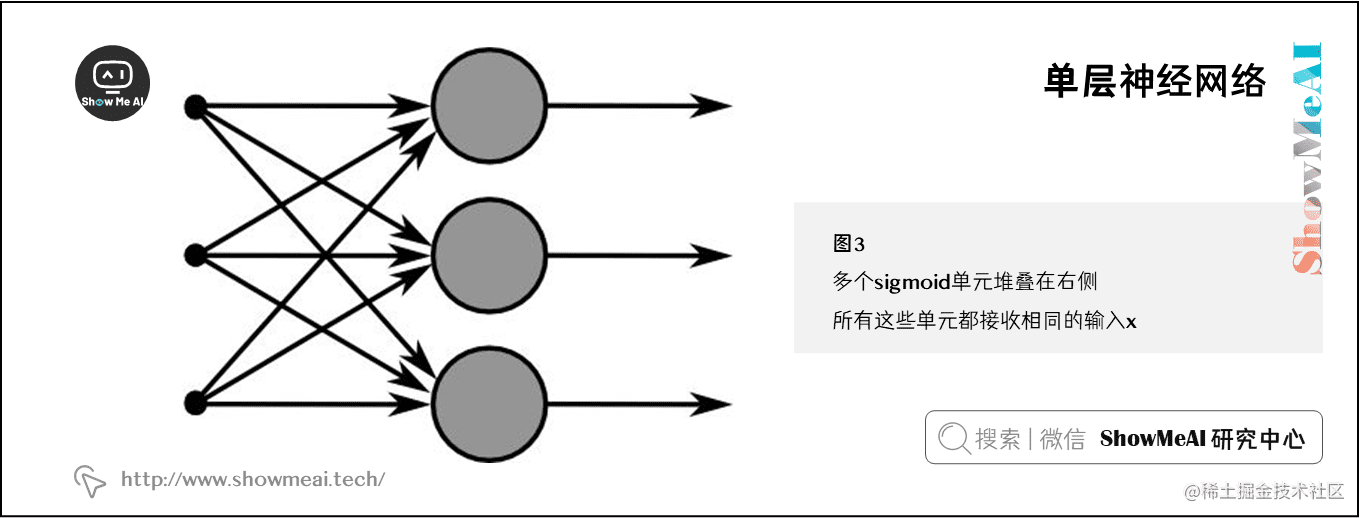$a_{1} =\frac{1}{1+exp(-(w^{(1)T}x+b_1))}$
$\vdots$
$a_{m} =\frac{1}{1+exp(-(w^{(m)T}x+b_m))}$

$\sigma(z) = \begin{bmatrix} \frac{1}{1+exp(z_1)} \\ \vdots \\ \frac{1}{1+exp(z_m)} \end{bmatrix}$
$b = \begin{bmatrix} b_{1} \\ \vdots \\ b_{m} \end{bmatrix} \in \mathbb{R}^{m}$
$W = \begin{bmatrix} -\;\;w^{(1)T}\;\;- \\ \cdots \\ -\;\;w^{(m)T}\;\;- \end{bmatrix} \in \mathbb{R}^{m\times n}$

$z=Wx+b$

$\begin{bmatrix} a_{1} \\ \vdots \\ a_{m} \end{bmatrix} = \sigma(z) = \sigma(Wx+b)$

## 1.3 前向与反向计算

Museums in Paris are amazing

$s=\mathbf{U}^{T}a=\mathbf{U}^{T}f(Wx+b)$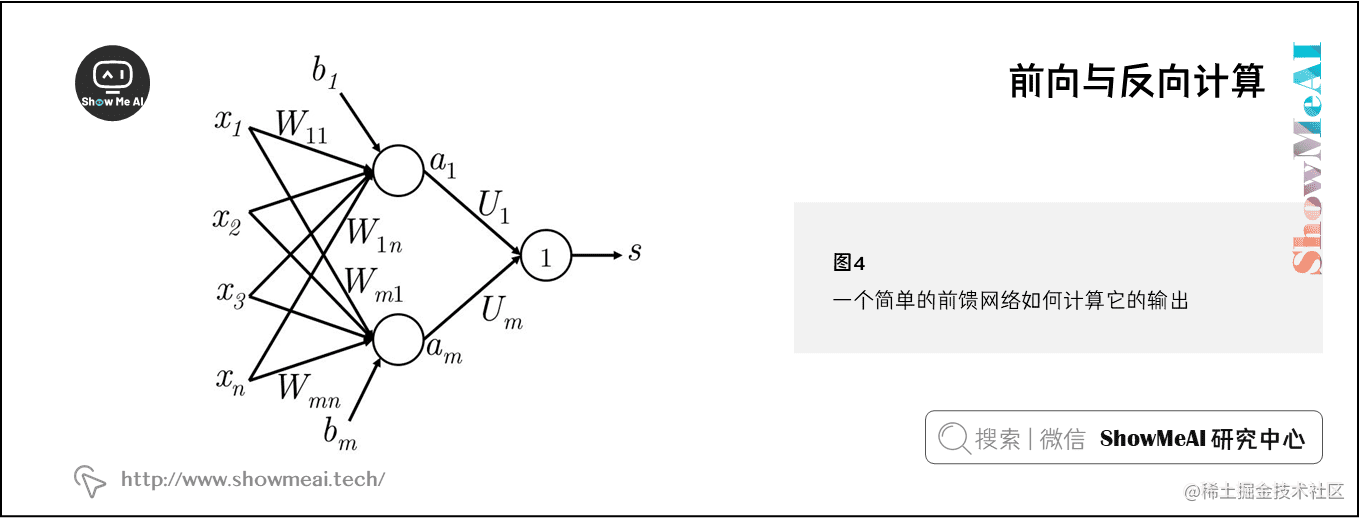## 1.4 合页损失

$minimize\;J=max\,(s_c-s,0)$

$minimize\;J=max\,(\Delta+s_c-s,0)$

$minimize\;J=max\,(1+s_c-s,0)$

$s_c=\mathbf{U}^{T}f(Wx_c+b)$
$s=\mathbf{U}^{T}f(Wx+b)$

❐ 最大边际目标函数通常与支持向量机一起使用

## 1.5 反向传播（单样本形态）

$\theta^{(t+1)}=\theta^{(t)}-\alpha\nabla_{\theta^{(t)}}J$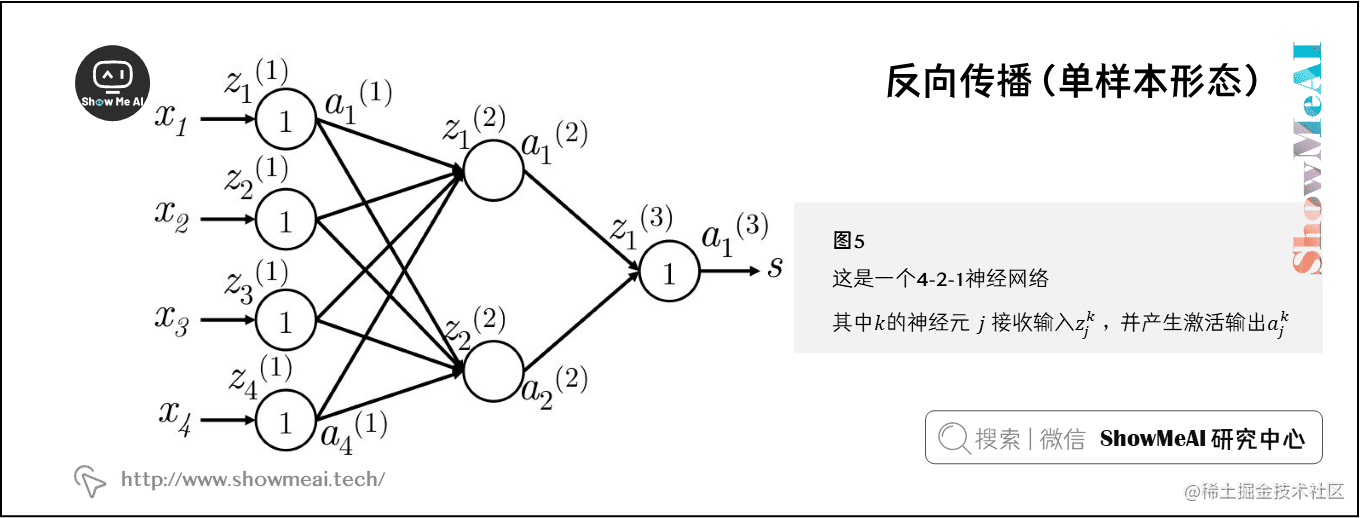• $x_i$ 是神经网络的输入
• $s$ 是神经网络的输出
• 每层(包括输入和输出层)的神经元都接收一个输入和生成一个输出。第 $k$ 层的第 $j$ 个神经元接收标量输入 $z_j^{(k)}$ 和生成一个标量激活输出 $a_j^{(k)}$
• 我们把 $z_j^{(k)}$ 计算出的反向传播误差定义为 $\delta_j^{(k)}$
• $1$ 层是输入层，而不是第 $1$ 个隐藏层。对输入层而言， $x_j=z_j^{(1)}=a_j^{(1)}$
• $W^{(k)}$ 是将第 $k$ 层的输出映射到第 $k+1$ 层的输入的转移矩阵，因此将这个新的符号用在上面1.3节中的例子 $W^{(1)}=W$$W^{(2)}=U$

$\frac{\partial J}{\partial s}=-\frac{\partial J}{\partial s_c}=-1$

\begin{aligned} \frac{\partial s}{\partial W_{ij}^{(1)}} &= \frac{\partial W^{(2)}a^{(2)}}{\partial W_{ij}^{(1)}}=\frac{\partial W_i^{(2)}a_i^{(2)}}{\partial W_{ij}^{(1)}}=W_i^{(2)}\frac{\partial a_i^{(2)}}{\partial W_{ij}^{(1)}} \\ \Rightarrow W_i^{(2)}\frac{\partial a_i^{(2)}}{\partial W_{ij}^{(1)}} &= W_i^{(2)}\frac{\partial a_i^{(2)}}{\partial z_i^{(2)}}\frac{\partial z_i^{(2)}}{\partial W_{ij}^{(1)}} \\ &= W_i^{(2)}\frac{f(z_i^{(2)})}{\partial z_i^{(2)}}\frac{\partial z_i^{(2)}}{\partial W_{ij}^{(1)}} \\ &= W_i^{(2)}f^{\prime}(z_i^{(2)})\frac{\partial z_i^{(2)}}{\partial W_{ij}^{(1)}} \\ &= W_i^{(2)}f^{\prime}(z_i^{(2)})\frac{\partial}{\partial W_{ij}^{(1)}}(b_i^{(1)}+a_1^{(1)}W_{i1}^{(1)}+a_2^{(1)}W_{i2}^{(1)}+a_3^{(1)}W_{i3}^{(1)}+a_4^{(1)}W_{i4}^{(1)}) \\ &= W_i^{(2)}f^{\prime}(z_i^{(2)})\frac{\partial}{\partial W_{ij}^{(1)}}(b_i^{(1)}+\sum_{k}a_{k}^{(1)}W_{ik}^{(1)}) \\ &= W_i^{(2)}f^{\prime}(z_i^{(2)})a_j^{(1)} \\ &= \delta_i^{(2)}\cdot a_j^{(1)} \end{aligned}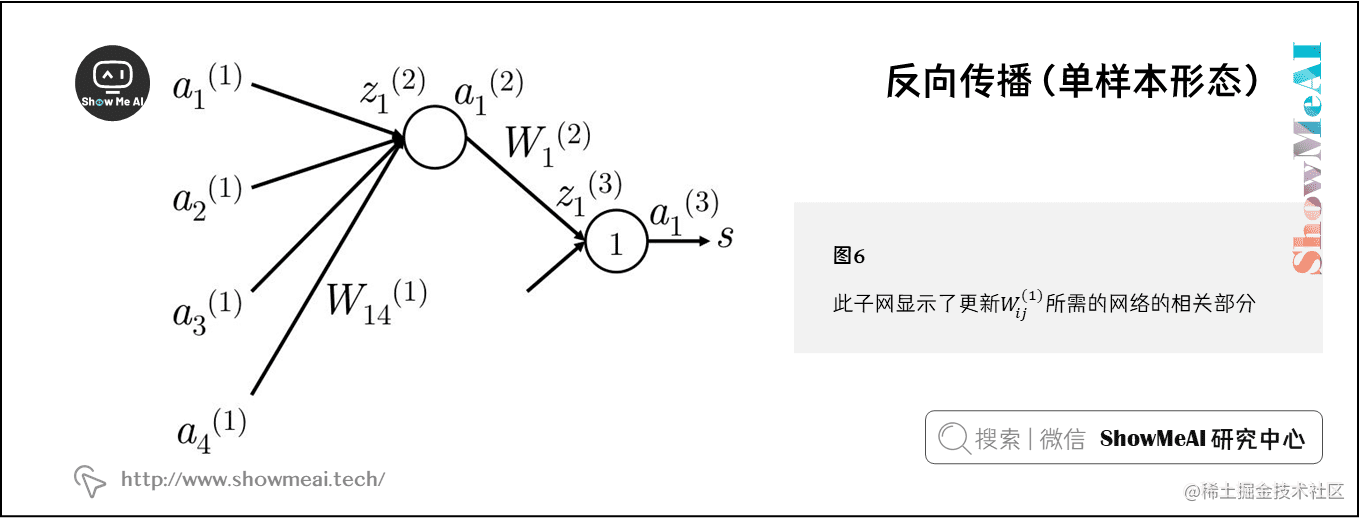• ① 我们从 $a_1^{(3)}$ 的1的误差信号开始反向传播
• ② 然后我们把误差与将 $z_1^{(3)}$ 映射到 $a_1^{(3)}$ 的神经元的局部梯度相乘。在这个例子中梯度正好等于1，则误差仍然为1。所以有 $\delta_1^{(3)}=1$
• ③ 这里误差信号1已经到达 $z_1^{(3)}$ 。我们现在需要分配误差信号使得误差的“公平共享”到达 $a_1^{(2)}$
• ④ 现在在 $a_1^{(2)}$ 的误差为 $\delta_1^{(3)}\times W_1^{(2)}=W_1^{(2)}$ (在 $z_1^{(3)}$ 的误差信号为 $\delta_1^{(3)}$ )。因此在 $a_1^{(2)}$ 的误差为 $W_1^{(2)}$
• ⑤ 与第2步的做法相同，我们在将 $z_1^{(2)}$ 映射到 $a_1^{(2)}$ 的神经元上移动误差，将 $a_1^{(2)}$ 与局部梯度相乘，这里的局部梯度为 $f'(z_1^{(2)})$
• ⑥ 因此在 $z_1^{(2)}$ 的误差是 $f'(z_1^{(2)})W_1^{(2)}$ ，我们将其定义为 $\delta_1^{(2)}$
• ⑦ 最后，我们通过将上面的误差与参与前向计算的 $a_4^{(1)}$ 相乘，把误差的“误差共享”分配到 $W_{14}^{(1)}$
• ⑧ 所以，对于 $W_{14}^{(1)}$ 的梯度损失可以计算为 $a_4^{(1)}f'(z_1^{(2)})W_1^{(2)}$

$\delta^{(k)}$$\delta^{(k-1)}$ 反向传播的一般步骤：

• ① 我们有从 $z_i^{(k)}$ 向后传播的误差 $\delta_i^{(k)}$ ，如下图所示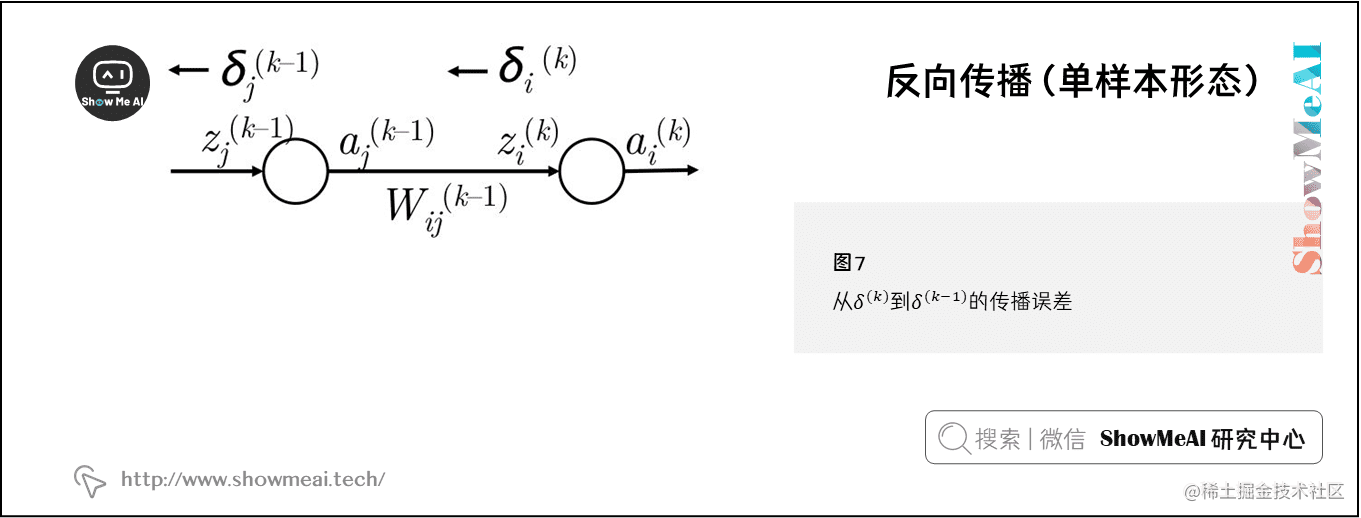• ② 我们通过把 $\delta_i^{(k)}$ 与路径上的权值 $W_{ij}^{(k-1)}$ 相乘，将这个误差反向传播到 $a_j^{(k-1)}$
• ③ 因此在 $a_j^{(k-1)}$ 接收的误差是 $\delta_i^{(k)}W_{ij}^{(k-1)}$
• ④ 然而， $a_j^{(k-1)}$ 在前向计算可能出下图的情况，会参与下一层中的多个神经元的计算。那么第 $k$ 层的第 $m$ 个神经元的误差也要使用上一步方法将误差反向传播到 $a_j^{(k-1)}$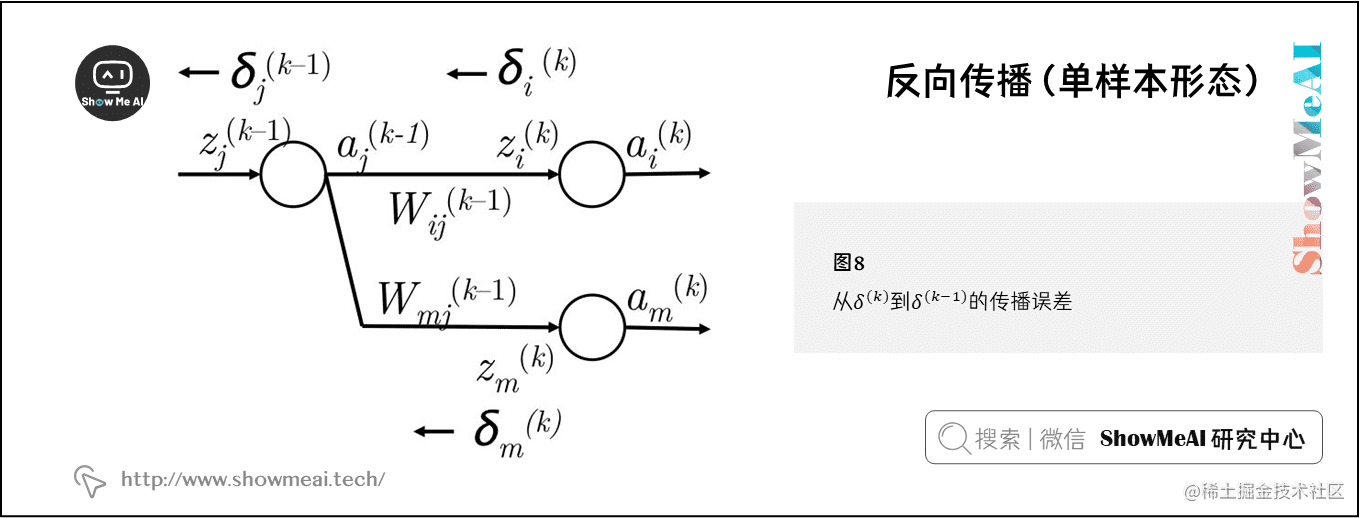• ⑤ 因此现在在 $a_j^{(k-1)}$ 接收的误差是 $\delta_i^{(k)}W_{ij}^{(k-1)}+\delta_m^{(k)}W_{mj}^{(k-1)}$
• ⑥ 实际上，我们可以把上面误差和简化为 $\sum_i\delta_i^{(k)}W_{ij}^{(k-1)}$
• ⑦ 现在我们有在 $a_j^{(k-1)}$ 正确的误差，然后将其与局部梯度 $f^{\prime}(z_j^{(k-1)})$ 相乘，把误差信息反向传到第 $k-1$ 层的第 $j$ 个神经元上
• ⑧ 因此到达 $z_j^{(k-1)}$ 的误差为 $f ^{\prime} (z_j^{(k-1)})\sum_i\delta_i^{(k)}W_{ij}^{(k-1)}$

## 1.6 反向传播（向量化形态）

$\nabla_{W^{(k)}} = \begin{bmatrix} \delta_1^{(k+1)}a_1^{(k)} & \delta_1^{(k+1)}a_2^{(k)} & \cdots \\ \delta_2^{(k+1)}a_1^{(k)} & \delta_2^{(k+1)}a_2^{(k)} & \cdots \\ \vdots & \vdots & \ddots \\ \end{bmatrix} = \delta^{(k+1)}a^{(k)T}$

$\delta_i^{(k)}=f^{\prime}(z_j^{(k)})\sum_i\delta_i^{(k+1)}W_{ij}^{(k)}$

$\delta_i^{(k)}=f^{\prime} (z^{(k)})\circ (W^{(k)T}\delta^{(k+1)})$

# 2.神经网络：技巧与建议

（本部分内容也可以参考ShowMeAI的对吴恩达老师课程的总结文章深度学习教程 | 深度学习的实用层面

## 2.1 梯度检查

$f^{\prime}(\theta)\approx \frac{J(\theta^{(i+)})-J(\theta^{(i-)})}{2\varepsilon }$

$f^{\prime}(\theta)\approx \frac{f(x+\varepsilon)-f(x)}{\varepsilon}$

• ① 我们需要考虑效率——每当我们想计算一个元素的梯度，需要在网络中做两次前向传播，这样是很耗费计算资源的。
• ② 很多大规模的神经网络含有几百万的参数，对每个参数都计算两次明显不是一个好的选择。
• ③ 在例如 SGD 这样的优化技术中，我们需要通过数千次的迭代来计算梯度，使用这样的方法很快会变得难以应付。

def eval_numerical_gradient(f, x):
"""
a naive implementation of numerical gradient of f at x
- f should be a function that takes a single argument
- x is the point (numpy array) to evaluate the gradient
at
"""

f(x) = f(x) # evaluate function value at original point
h = 0.00001

# iterate over all indexes in x
it = np.nditer(x, flags=['multi_index',

while not it.finished:

# evaluate function at x+h
ix = it.multi_index
old_value = x[ix]
x[ix] = old_value + h # increment by h
fxh_left = f(x) # evaluate f(x + h)
x[ix] = old_value - h # decrement by h
fxh_right = f(x) # evaluate f(x - h)
# restore to previous value (very important!)
x[ix] = old_value

# compute the partial derivative
# the slope
grad[ix] = (fxh_left - fxh_right) / (2 * h)
it.iternext() # step to next dimension

## 2.2 正则化

$J_{R}=J+\lambda\sum_{i=1}^{L}\left \| W^{(i)} \right \| _F$

❐ 矩阵 $U$ 的 Frobenius 范数的定义： $\left \| U \right \| _F=\sqrt{\sum_i \sum_{l} U_{i l}^{2}}$

• $\lambda$ 的值太大会令很多权值都接近于 $0$ ，则模型就不能在训练集上学习到有意义的东西，经常在训练、验证和测试集上的表现都非常差。
• $\lambda$ 的值太小，会让模型仍旧出现过拟合的现象。

❐ Dropout 实际上是通过在每次迭代中忽略它们的权值来实现“冻结”部分 unit 。这些“冻结”的 unit 不是把它们设为 $0$ ，而是对于该迭代，网络假定它们为 $0$ 。“冻结”的 unit 不会为此次迭代更新

## 2.3 随机失活Dropout

Dropout 是一个非常强大的正则化技术，是 Srivastava 在论文 《Dropout: A Simple Way to Prevent Neural Networks from Overﬁtting》中首次提出，下图展示了 Dropout 如何应用在神经网络上。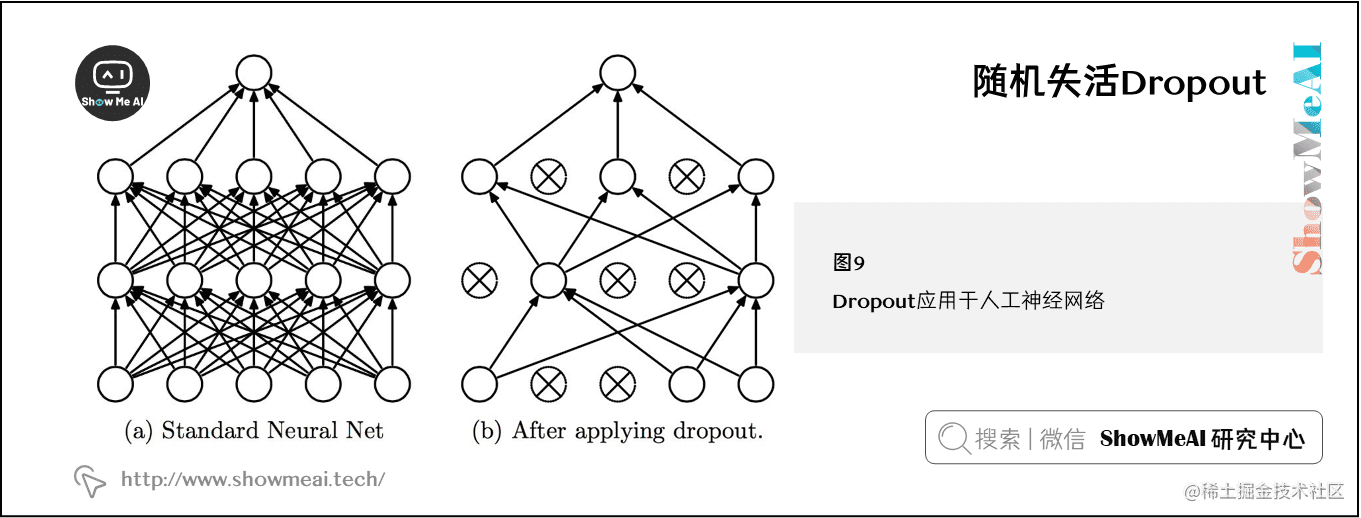### 1) Dropout内容补充

• 目的：缓解过拟合问题，一定程度上达到正则化的效果

• 效果：减少下层节点对其的依赖，迫使网络去学习更加鲁棒的特征

### 3) 贝叶斯学习的解释

\begin{aligned} \mathbb{E}_{q(\theta)}[y] &=\int_{q} f(\mathbf{x}, \theta) q(\theta) d \theta \\ & \approx \frac{1}{M} \sum_{m=1}^{M} f\left(\mathbf{x}, \theta_m\right) \end{aligned}

### 4) RNN中的变分Dropout (Variational Dropout)

Dropout一般是针对神经元进行随机丢弃，但是也可以扩展到对神经元之间的连接进行随机丢弃，或每一层进行随机丢弃。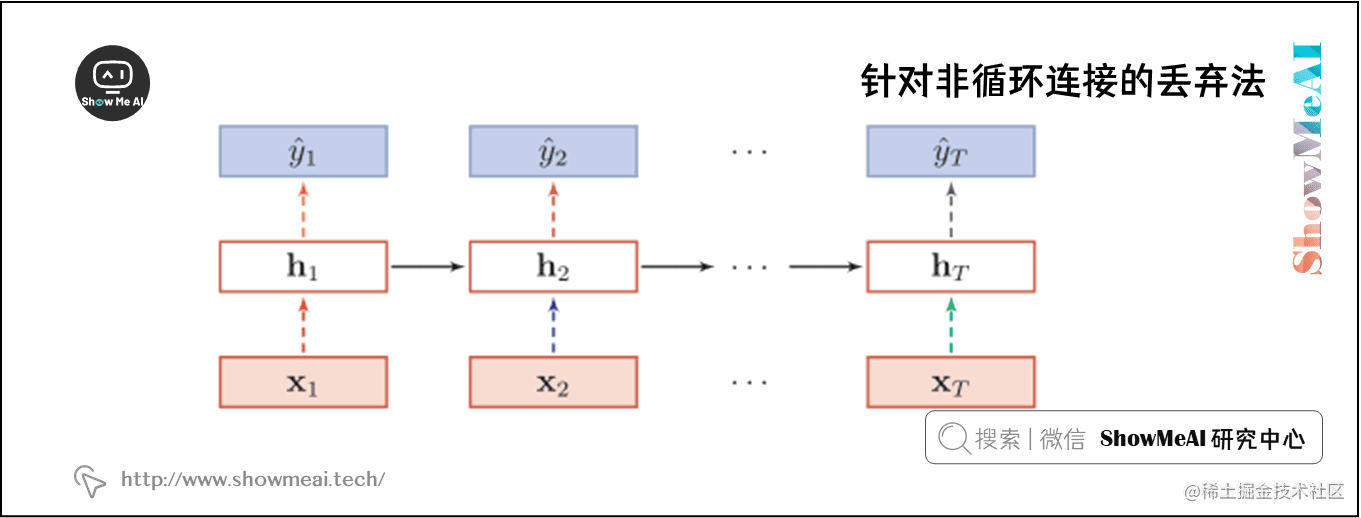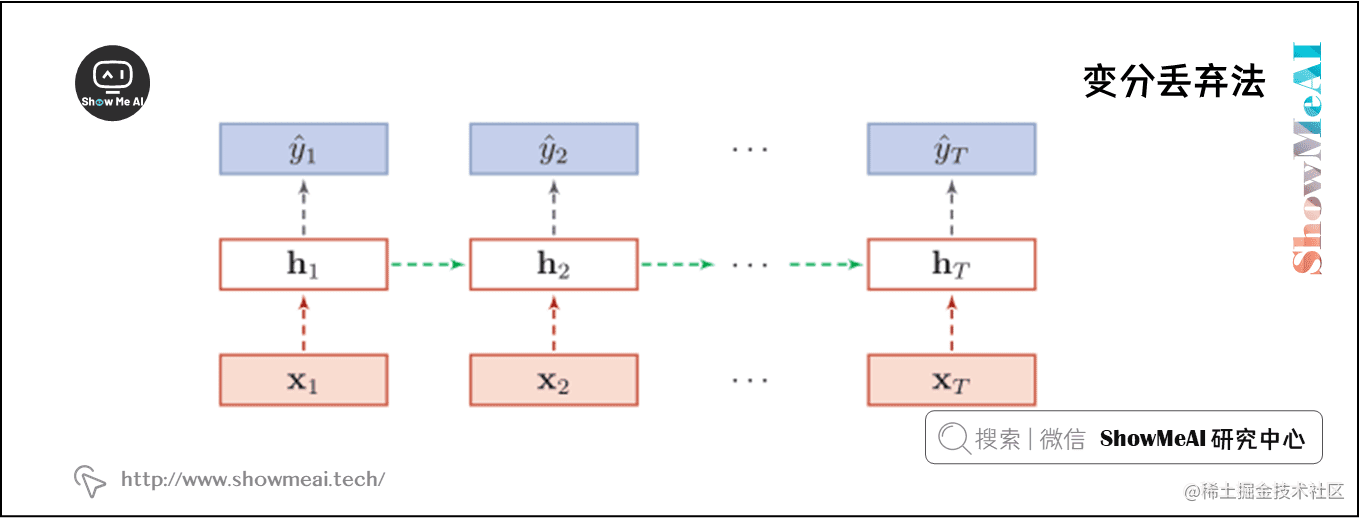## 2.4 神经元激活函数

### 1) Sigmoid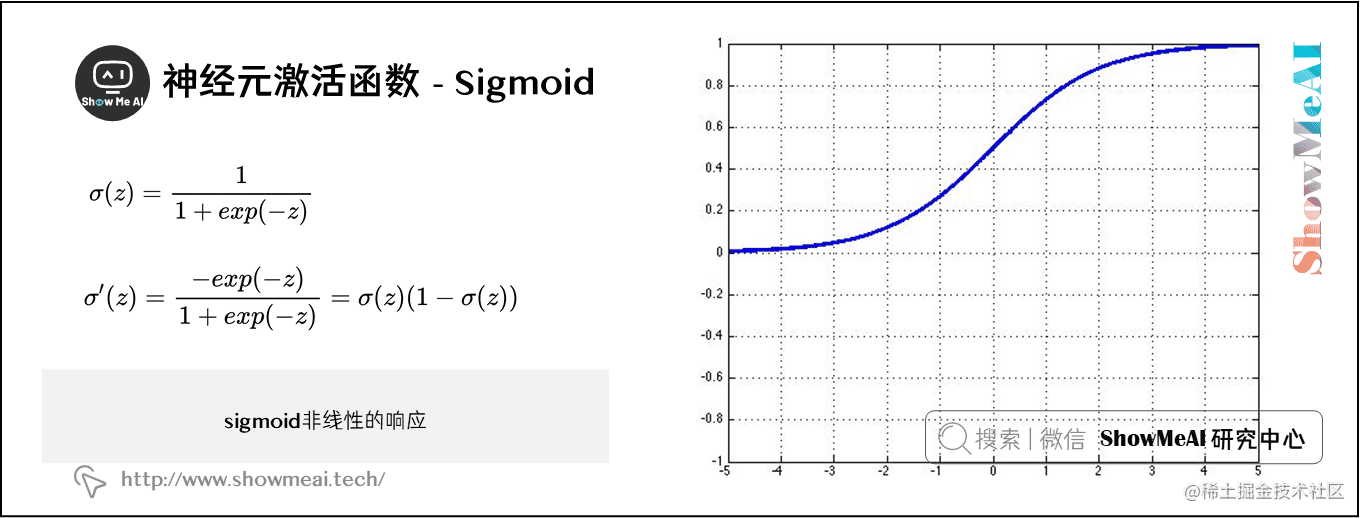$\sigma(z)=\frac{1}{1+exp(-z)}$

$\sigma(z)$ 的梯度为：

$\sigma^{\prime}(z)=\frac{-exp(-z)}{1+exp(-z)}=\sigma(z)(1-\sigma(z))$

### 2) tanh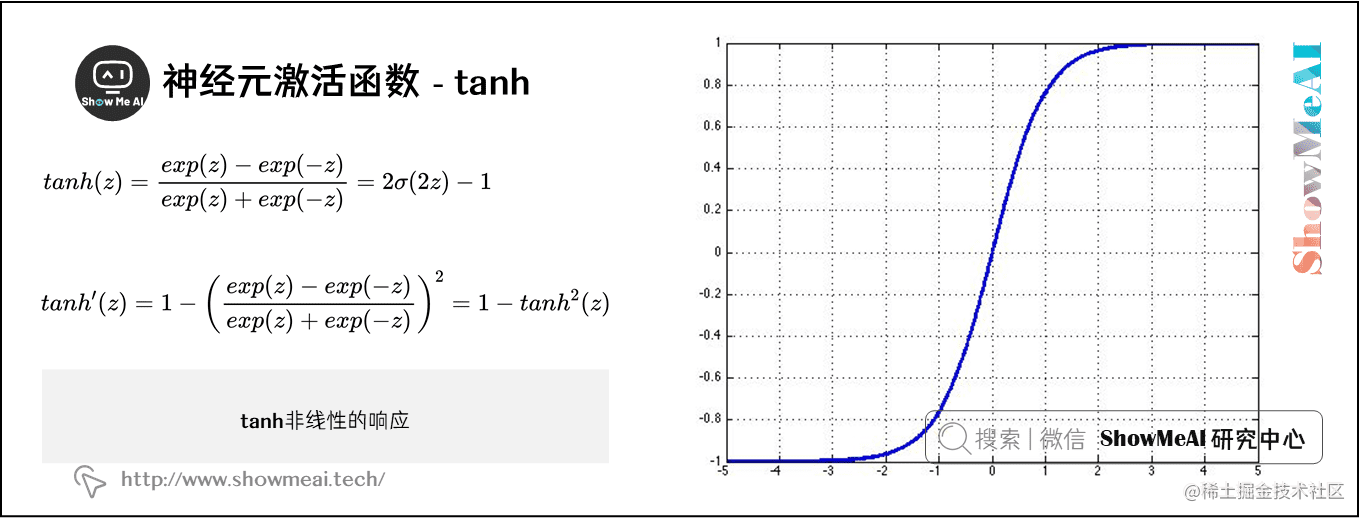tanh函数是sigmoid函数之外的另一个选择，在实际中它能更快地收敛。tanh和sigmoid的主要不同在于tanh的输出范围在-1到1，而sigmoid的输出范围在0到1。

$tanh(z)=\frac{exp(z)-exp(-z)}{exp(z)+exp(-z)}=2\sigma(2z)-1$

$tanh(z)$的梯度为：

$tanh^{\prime}(z)=1-\bigg(\frac{exp(z)-exp(-z)}{exp(z)+exp(-z)}\bigg)^{2}=1-tanh^{2}(z)$

### 3) hard tanh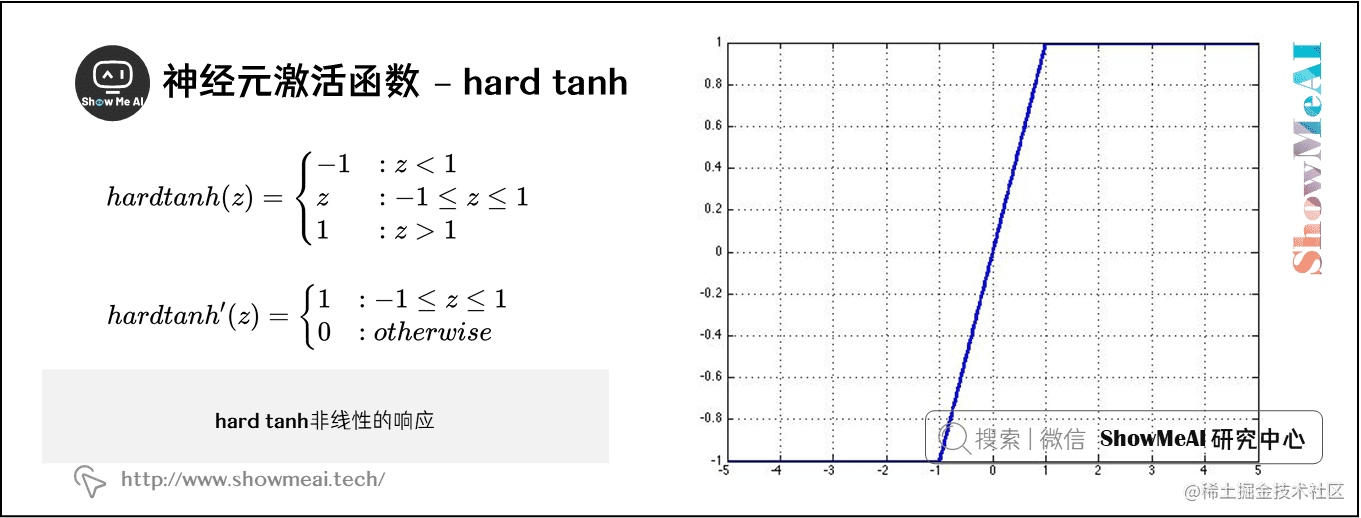hardtanh激活函数为：

\begin{aligned} hardtanh(z) = \begin{cases} -1& :z<1\\ z & :-1\le z \le 1 \\ 1 & :z>1 \end{cases} \end{aligned}

hardtanh这个函数的微分也可以用分段函数的形式表示：

\begin{aligned} hardtanh ^{\prime}(z) &= \begin{cases} 1 & :-1\le z \le 1 \\ 0 & :otherwise \end{cases} \end{aligned}

### 4) soft sign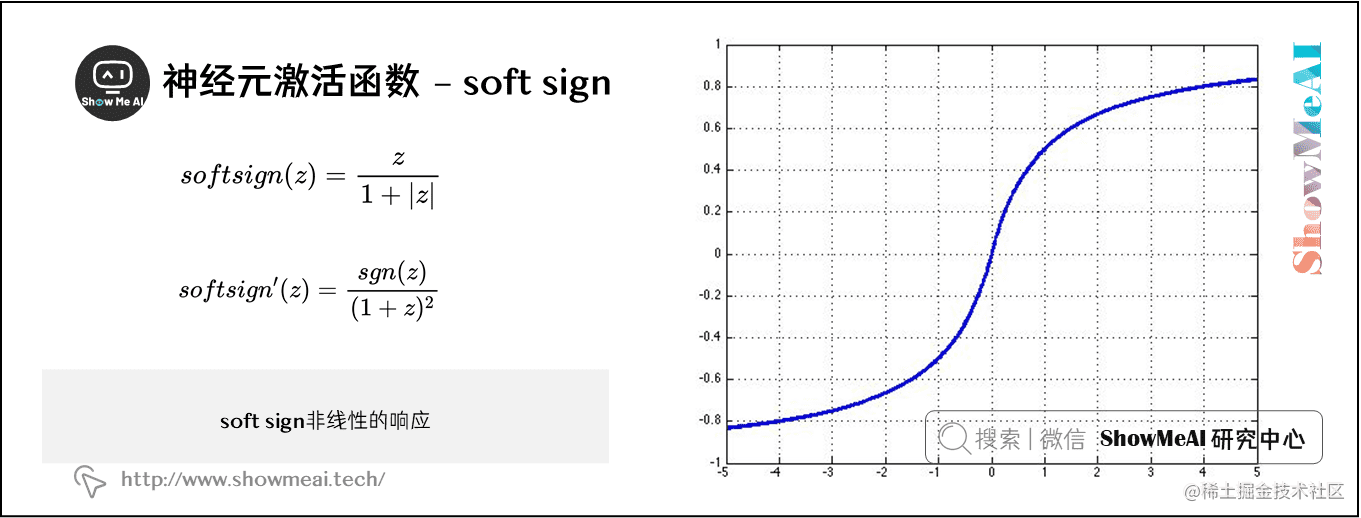soft sign函数是另外一种非线性激活函数，它可以是tanh的另外一种选择，因为它和hard clipped functions 一样不会过早地饱和：

$softsign(z)=\frac{z}{1+ \left | z \right |}$

soft sign函数的微分表达式为：

$softsign^{\prime}(z)=\frac{sgn(z)}{(1+z)^{2}}$

### 5) ReLU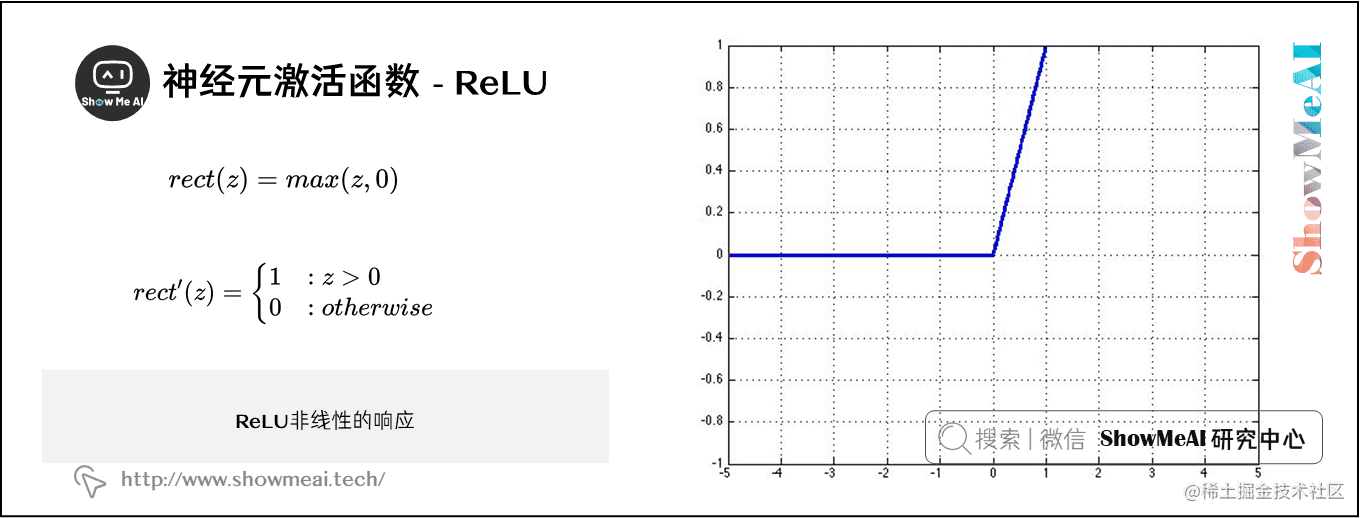ReLU ( Rectiﬁed Linear Unit )函数是激活函数中的一个常见的选择，当 $z$ 的值特别大的时候它也不会饱和。在计算机视觉应用中取得了很大的成功：

$rect(z)=max(z,0)$

ReLU函数的微分是一个分段函数：

\begin{aligned} rect^{\prime}(z) &= \begin{cases} 1 & :z > 0 \\ 0 & :otherwise \end{cases} \end{aligned}

### 6) Leaky ReLU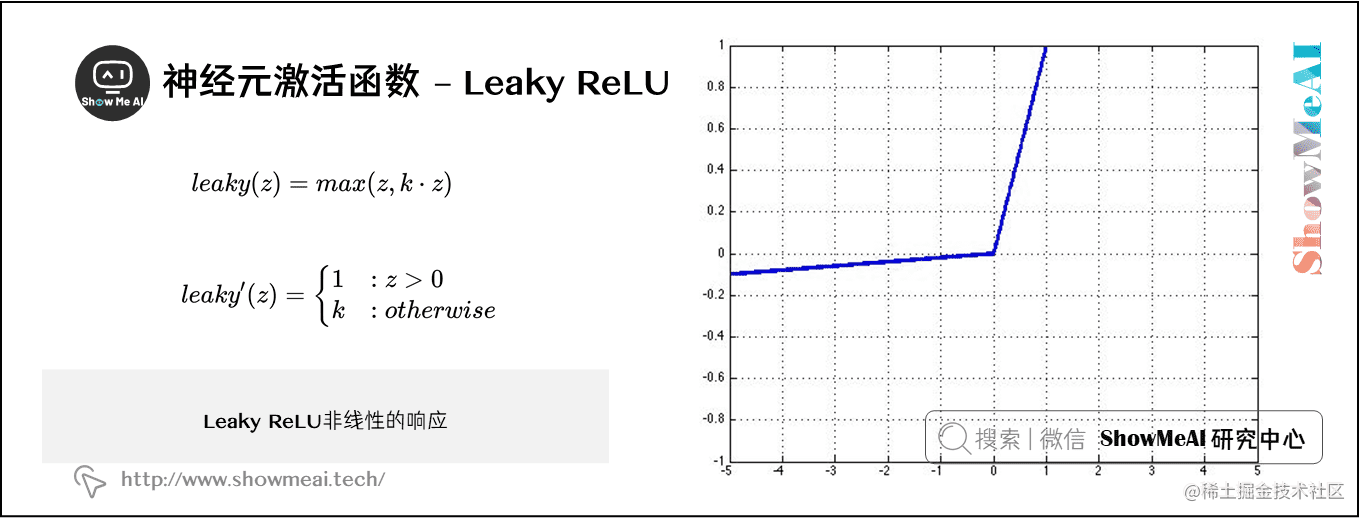$leaky(z)=max(z, k\cdot z)$

leaky ReLU函数的微分是一个分段函数：

\begin{aligned} leaky ^{\prime} (z) &= \begin{cases} 1 & :z > 0 \\ k & :otherwise \end{cases} \end{aligned}

## 2.6 参数初始化

$W\sim U\bigg[-\sqrt{\frac{6}{n^{(l)}+n^{(l+1)}}},\sqrt{\frac{6}{n^{(l)}+n^{(l+1)}}}\;\bigg]$

## 2.7 学习策略

$\theta^{new}=\theta^{old}-\alpha\nabla_{\theta}J_{t}(\theta)$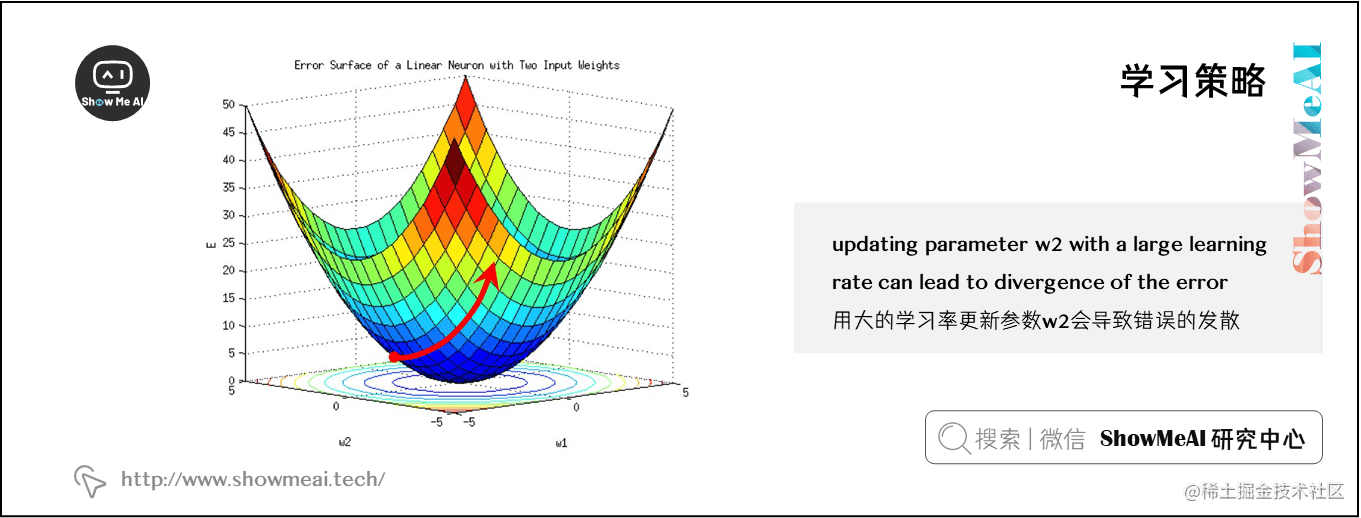$\alpha(t)=\frac{\alpha_0\tau}{max(t,\tau)}$

## 2.8 带动量的优化更新（Momentum）

（神经网络优化算法也可以参考ShowMeAI的对吴恩达老师课程的总结文章深度学习教程 | 神经网络优化算法

# Computes a standard momentum update
# on parameters x
v = mu * v - alpha * grad_x
x += v

## 2.9 自适应优化算法

（神经网络优化算法也可以参考ShowMeAI的对吴恩达老师课程的总结文章深度学习教程 | 神经网络优化算法

$\theta_{t,i}=\theta_{t-1,i}-\frac{\alpha}{\sqrt{\sum_{\tau=1}^{t}g_{\tau,i}^{2}}} g_{t,i} \\ where \ g_{t,i}=\frac{\partial}{\partial\theta_i^{t}}J_{t}(\theta)$

# Assume the gradient dx and parameter vector x
cache += dx ** 2
x += -learning_rate * dx / np.sqrt(cache + 1e-8)

# Update rule for RMS prop
cache = decay_rate * cache + (1 - decay_rate) * dx ** 2
x += -learning_rate * dx / (np.sqrt(cache) + eps)

m = beta * m + (1 - beta1) * dx
v = beta * v + (1 - beta2) * (dx ** 2)
x += -learning_rate * m / (np.sqrt(v) + eps)

# 斯坦福 CS224n 课程带学详解